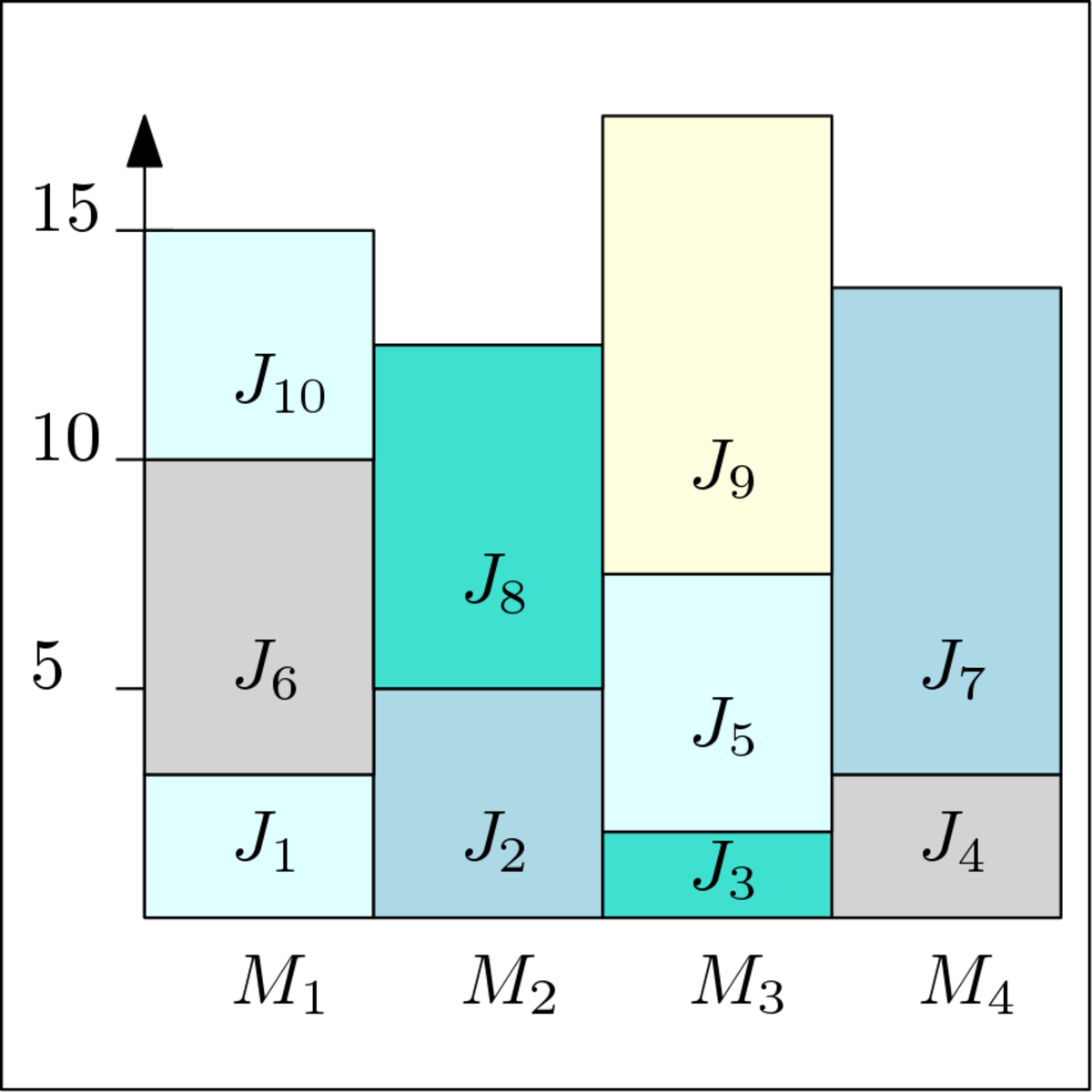# Approximation Algorithms

EIT Digital

Many real-world algorithmic problems cannot be solved efficiently using traditional algorithmic tools, for example because the problems are NP-hard. The goal of this course is to become familiar with important algorithmic concepts and techniques needed to effectively deal with such problems. These techniques apply when we don't require the optimal solution to certain problems, but an approximation that is close to the optimal solution. We will see how to efficiently find such approximations. Prerequisites: In order to successfully take this course, you should already have a basic knowledge of algorithms and mathematics. Here's a short list of what you are supposed to know: - O-notation, Ω-notation, Θ-notation; how to analyze algorithms - Basic calculus: manipulating summations, solving recurrences, working with logarithms, etc. - Basic probability theory: events, probability distributions, random variables, expected values etc. - Basic data structures: linked lists, stacks, queues, heaps - (Balanced) binary search trees - Basic sorting algorithms, for example MergeSort, InsertionSort, QuickSort - Graph terminology, representations of graphs (adjacency lists and adjacency matrix), basic graph algorithms (BFS, DFS, topological sort, shortest paths) The material for this course is based on the course notes that can be found under the resources tab. We will not cover everything from the course notes. The course notes are there both for students who did not fully understand the lectures as well as for students who would like to dive deeper into the topics. The video lectures contain a few very minor mistakes. A list of these mistakes can be found under resources (in the document called "Errata"). If you think you found an error, report a problem by clicking the square flag at the bottom of the lecture or quiz where you found the error.

Certificate Available ✔#### Introducción a la inteligencia artificial

Este Programa especializado está dirigido a personas con interés en conocer más sobre los diversos desarrollos que han sido generados en décadas recientes en...

#### Data Structures and Algorithms (II)

Tsinghua University

By learning this course, you will get a comprehensive grasp of stack, queue, binary tree, graph and BST structures and algorithms, as well as their applications....

#### Operations Research (1): Models and Applications

National Taiwan University

Operations Research (OR) is a field in which people use mathematical and engineering methods to study optimization problems in Business and Management, Economics,...

#### 数据结构基础

Peking University